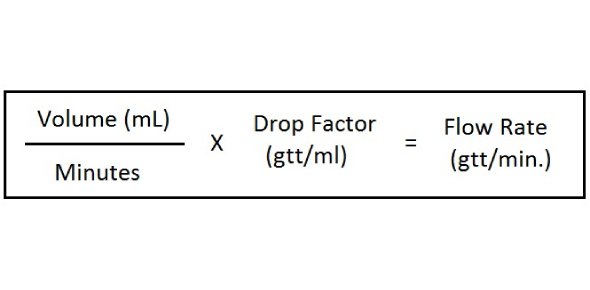# IV Drip Rate Formula: Calculation Practice Quiz!

15 Questions | Attempts: 19158SettingsAre you pursuing a nursing course? IV drip-rate formula: calculation practice quiz is designed for practicing nursing exam with this module. This quiz will test your knowledge on how to solve IV flow rate drip. In nursing school, you will have to learn how to calculate how much intravenous medication will be given via a flow rate. Flow rates are measured in mL/hr (milliliters per hour). Although in the job setting, most IV pumps will automatically calculate this, you will need to know how to double-check the IV pump to ensure the right amount of drug is being infused.

• 1.
Ordered: 500 mg ampicillin in 100mL NS to infuse over 30 minutes. What is the flow rate per hour?
• A.

20 mL

• B.

50 mL

• C.

100 ml

• D.

200 mL

• 2.
Ordered: 500 mL 5% Dextrose 0.45% NS over 3 hours. Find the flow rate per hour.
• A.

125 mL

• B.

167 mL

• C.

250 mL

• D.

500 mL

• 3.
Ordered: 1000 mL LR over 6 hours.  Find the flow rate in mL per hour.
• A.

17 mL

• B.

167 mL

• C.

175 mL

• D.

217 mL

• 4.
Find the flow rate in mL per hour. 300 mL NS over 2 H.
• A.

150 mL

• B.

200 mL

• C.

300 mL

• D.

600 Ml

• 5.
Find the flow rate in mL per hour. 3000 mL 0.45% over 24 H.
• A.

75 mL

• B.

100 mL

• C.

125 mL

• D.

150 mL

• 6.
Find the flow rate in mL per hour. 1500 mL RL over 12 hours.
• A.

83

• B.

94

• C.

100

• D.

125

• 7.
Find the flow rate in mL per hour. 40 mEq KCL in 100 mL NS over 45 minutes.
• A.

66 mL

• B.

75 mL

• C.

125 mL

• D.

133 mL

• 8.
Find the flow rate in mL per hour. 1000 mL NS over 12 hours.
• A.

83

• B.

94

• C.

100

• D.

125

• 9.
Find the flow rate in mL per hour. 20 mEq KCL in 50 mL NS over 30 minutes.
• A.

94

• B.

100

• C.

125

• D.

83

• 10.
Find the flow rate in mL per hour. 750 mL NS over 8 hours.
• A.

83

• B.

94

• C.

100

• D.

125

• 11.
Calculate the flow rate for manually regulated IV using the drops per minute. Ordered: 1000 mL NS over 24 hours, tubing is 20 gtt/mL. Write the number only of the drops per minute.
• 12.
Calculate the flow rate for manually regulated IV using the drops per minute. Ordered: 250 mL D5W over 3 hours, tubing is 10 gtt/mL. Write the number only of the drops per minute.
• 13.
Calculate the flow rate for manually regulated IV using the drops per minute. Ordered: 40 mEq KCL in 100 mL NS over 40 minutes, tubing is 20gtt/mL. Write the number only of the drops per minute.
• 14.
Calculate the flow rate for manually regulated IV using the drops per minute. Ordered: 1500 mL 0.45% NS over 12 hours, tubing is 15 gtt/mL. Write the number only of the drops per minute.
• 15.
Calculate the flow rate for manually regulated IV using the drops per minute. Ordered: 500 mL NS over 8hours, tubing is 15 gtt/mL. Write the number only of the drops per minute.

## Related TopicsBack to top
×

Wait!
Here's an interesting quiz for you.